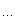# 聚星亭

posts - 74, comments - 166, trackbacks - 0, articles - 0
C++博客 :: 首页 :: 新随笔 :: 联系 :: 聚合:: 管理
[声明] 本文引用与：GameRes 游戏开发网

前一段在http://www.allaboutprogram.com/上看到有关于排序方法的时间复杂度的研究，说的是在一般情况下，最好的时间复杂度是 O( n*LOG(n) )， 而在特定的情况下，比如要排序的数据是整数，而且比较集中，也可以简化为 O(n)。

后来我也给abp写了封信，说明了一下对于一般的整数（int 或者unsigned int），也可以进行复杂度为 O(n)的排序。我后来给了他一个我写的STL的版本，实现了复杂度为O(n)的int排序。但实测起来，至少在n = 1000*1024的时候，还是比STL的sort 要慢。

应abp的要求，我写了这篇文章，作为复杂度为O(n)的这种排序 ---- Radix Sort的介绍。今天，我在网上找到一小段代码，和我写的几乎一样，不过没有用STL，实测性能在n =1024*1000的时候，VC 6 上 release 模式，排序时间：(tick)

100*1024                 20                      20
1000*1024              250                    320
4000*1024              991                  1513
10000*1024           2093                 3606

考虑一种简单情况，如果你给一些unsigned char 排序，除了教科书上的很多方法外，还有一种简单的。可以这样考虑。试想排一系列的unsigned char, 值从0~ 255 ， 放在 u_char data_array[ARRAY_SIZE] 中，分配一个数组 u_char membuffer;

for(int i=0;i<255;i++
membuffer[i]
=0
for(int i=0;i<ARRAY_SIZE;i++
membuffer[data_array[i]]
++

这样，就把数字填到了membuffer内部，然后，比如这个是输出的数组

u_char sorted_array[ARRAY_SIZE];

int k=0

for(int i=0;i<255;i++

for(int j=0;j<membuffer[i]; j++
sorted_array[k
++]=i;

图例：在256个entry的数组中，可能产生一系列的重复值。如下：

每个entry中实际并不记录值，而是记录重复的次数。

1   3 4 5   71      4

4

这样，就把n = ARRAY_SIZE 的unsigned char 进行了排序。

那么，如何扩展到对于一般的整数进行排序呢？可以这样考虑。一个 int,是由　４个　char 组成（在32-bit int的系统上）在 Little Endian　字节顺序（ Least Siginficant Byte first , 比如 80x86架构　）下， <- low memory address                       high memory address ->
char 0    char 1    char 2    char 3

char 0 就是 0x78
char 1 就是 0x56
char 2 就是 0x34
char 3 就是 0x12

如果我们用最低位字节　（char 0）来作为 sort key对int 排序，然后使用次低做sort key, 然后用次高，最后用最高字节排序，实际的结果就是对整个int 进行了排序。

下面的a, b, c, d指int 的不同char, 让我们进行一下升序排序(ascending sort)

abcd
bacd
dcba
caba
bbac

dcba
caba
bbac
abcd
bacd

bbac
dcba
caba
abcd
bacd

caba
bacd
bbac
abcd
dcba

abcd

bacd
bbac
caba
dcba

using namespace std;

typedef vector
<int>  HASH;
typedef unsigned
char  u_char;

HASH  hashtable[
256];  //256 entries hash table

const int array_size=1024*4000

void SortInt( int * data,  int size);

void SortIntByChar(int * data,  int size, int pos);

inline u_char GetHashvalue(
int  value, int pos)

return ((u_char*)&value)[pos];

void SortIntByChar(int * data,  int size, int pos)

int i;

//clear hashtable
for(i=0;i<256++i)
{
hashtable[i].clear();
}

for(i=0;i< size; ++i)
{
hashtable[GetHashvalue(data[i],  pos)].push_back(data[i]);
}

//output int
int k=0

if(pos!=3
{

for(i=0;i< 256++i)
{

for(int j=0;j< hashtable[i].size(); ++j)
{
data[k
++]=hashtable[i][j];
}
}
}

else  //most significant byte
{

for(i=128;i< 256++i)
{

for(int j=0;j< hashtable[i].size(); ++j)
{
data[k
++]=hashtable[i][j];
}
}

for(i=0;i< 128++i)
{

for(int j=0;j< hashtable[i].size(); ++j)
{
data[k
++]=hashtable[i][j];
}
}
}

void SortInt( int * data,  int size)

SortIntByChar(data, size,
0);
SortIntByChar(data, size,
1);
SortIntByChar(data, size,
2);
SortIntByChar(data, size,
3);

这里的方法，和前面说的最简单的并没有太大不同，不同的是，因为int是无法装入char的数组的，所以，使用了一个vector<int> ，为256个entry中的任何一个。这样，就把int的值装入了。

经过测试，这个效果并不太好，对于n=1000*1024下为500 ticks ，比 STL Sort 的　320 ticks要慢。那么，前面的250 ticks 如何得来的呢？这就是有关优化的问题。使用了STL，所以慢。因为在 clear()　和push_back（）中，作了过多你在这里不关心的事情。这里给出上面测试用的快速版本，未使用STL

void radix (int bytelong N, const long *source, long *dest)

long count;

long index;

memset (count,
0sizeof (count));

for ( int i=0; i<N; ++i ) ++count[((source[i])>>(byte*8))&0xff];

if(byte!=3
{
index[
0]=0

for ( i=1; i<256++i ) index[i]=index[i-1]+count[i-1];
}

else
{
index[
128]=0

for ( i=128+1; i<256++i )  index[i]=index[i-1]+count[i-1];

index[
0]=index+count;

for ( i=1; i<128++i ) index[i]=index[i-1]+count[i-1];
}

for ( i=0; i<N; ++i ) dest[index[((source[i])>>(byte*8))&0xff]++= source[i];

void radixsort (long *source, long *temp, long N)

0, N, source, temp);
1, N, temp, source);
2, N, source, temp);
3, N, temp, source);

不同的是，他多用了一个index数组来记录每一个entry的开始的对应于全体数据的index. 这样，就不必使用STL 的vector。

图二是IEEE 754 规范中单精度 float的格式：即VC 中的float。

在这里只讨论float，不讨论double。最高位(bit 31) 是符号位，如果为1，则表示为负，为0则为非负。Exp 有8位，是表示指数部分的，Significand 是小数部分。在把十进制表示的浮点数生成IEEE754各部分的时候，还有一些normalize 等操作，比较麻烦。因为这里主要讨论排序，所以不仔细说了。

负数小于正数，正数的绝对值越大值越大，负数相反。对于正数，指数大的肯定大。相同的指数，尾数大的更大。这样，也可以强行比较字节，从低字节开始比。可是第三个字节的最高位(bit23)有1位实际上是exp的最低位。

可以这样想，假设 A 和B 是两个正的float，A. bit 23代表A的第23位，那么：

Title

if ( A . bit 23 > B. bit 23 ) , then A >B 成立

if(A.bit 23< B. bit 23) , then A<B 成立。

那么，把指数的最低位放到和尾数一起组成的字节比较仍然可以比出浮点数的大小来。最后一次比较，最高字节的最高位是符号位。这可以特别处理。所以，比较浮点数和比较int 并没有太大的不同，就是有些符号处理的问题要注意。

Pierre Terdiman 给出了排序float的算法。测试结果和STL排序float比较一下，如下：

N                       Radix Sort (float)         STL Sort (float
100*1024                30                                  30
1000*1024             641                                431
4000*1024            2334                             1903
10000*1024          5788                             5498

（图三）

可以看出，至少在n=10000*1024的范围里，Pierre Terdiman 的Radix Sort 方法还是比 STL sort 要慢。但我测了一下Pierre Terdiman 的int 排序，也是比STL sort慢。推测可能是他的算法优化不够。从道理上讲，的确是O(n) 的复杂度，就是N 不是充分大的时候体现不出来。

我曾看过在一个Nvidia 的工具的source code，据称是最好的float 排序，但现在不在手边。等我找到了也加上来。不同的source code的算法倒是基本上一样，就是优化的程度不同。但小小的优化带来的结果也可能是显著的。

列出我写的整数排序方法，
Nils Pipenbrinck aka 的整数排序方法,
和 Pierre Terdiman的整数和浮点排序方法。